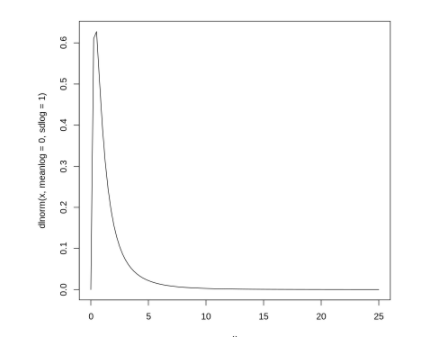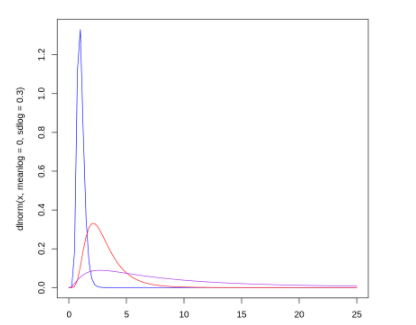# How to Plot a Log Normal Distribution in R

• Difficulty Level : Medium
• Last Updated : 28 Mar, 2022

In this article, we are going to see how to plot log-normal distribution in R Programming Language. A log-normal distribution is a continuous probability distribution of a random variable whose logarithm is normally distributed. In probability, if the random variable X is log-normally distributed, then Y = ln(X) has a normal distribution.

To plot the log-normal distribution we would require two functions namely dlnorm() and curve().

dlnorm(x, meanlog = 0, sdlog = 1)

Parameters:

• x – vector of quantiles
• meanlog – mean of the distribution on the log scale with a default value of 0.
• sdlog – standard deviation of the distribution on the log scale with default values of 1.

curve(expr, from = NULL, to = NULL)

Parameters:

• function – The name of a function, or a call or an expression written as a function of x which will evaluate to an object of the same length as x.
• from – the start range over which the function will be plotted.
• to – the end range over which the function will be plotted.

### Example 1:

In the first example let us plot a log-normal distribution using mean 0 and standard deviation 1 over a range of 0 to 25 using curve and dlnorm function.

## R

 `curve``(``dlnorm``(x, meanlog=0, sdlog=1), from=0, to=25)`

Output:### Example 2:

We know the fact that by default the mean and standard deviation values is 0 and 1 respectively, so we can plot the above function without specifying the meanlog and sd log parameters, the result is going to be the same.

## R

 `curve``(``dlnorm``(x), from=0, to=25)`

Output:### Example 3:

We can also draw multiple log-normal distributions with different mean, std. dev and the range by specifying different colors to each distribution as shown below.

## R

 `curve``(``dlnorm``(x, meanlog=0, sdlog=.3),``      ``from=0, to=25, col=``'blue'``)`` ` `curve``(``dlnorm``(x, meanlog=1, sdlog=.5),``      ``from=0, to=25, col=``'red'``, add=``TRUE``)`` ` `curve``(``dlnorm``(x, meanlog=2, sdlog=1), ``      ``from=0, to=25, col=``'purple'``, add=``TRUE``)`

Output:My Personal Notes arrow_drop_up